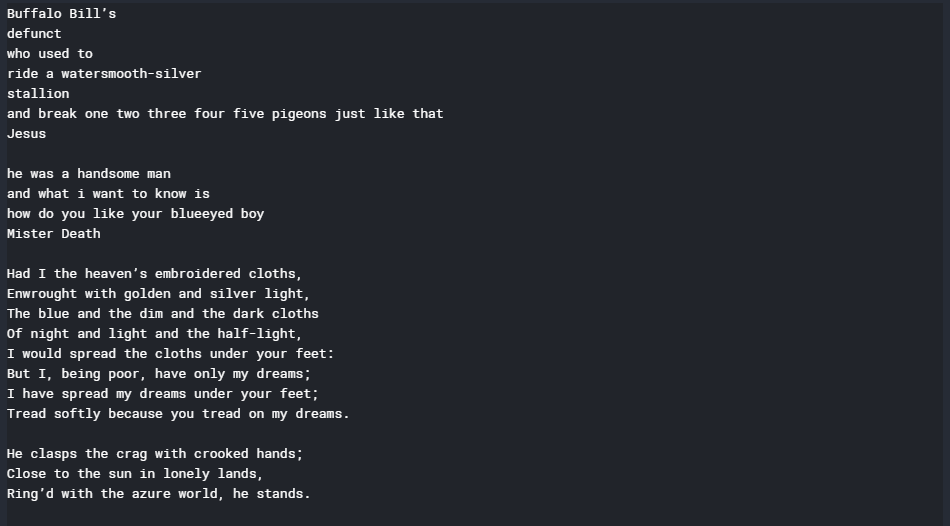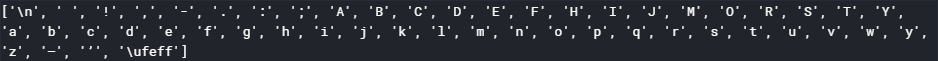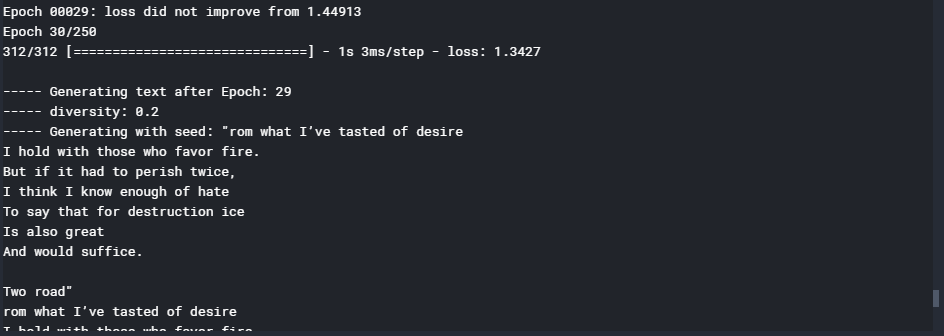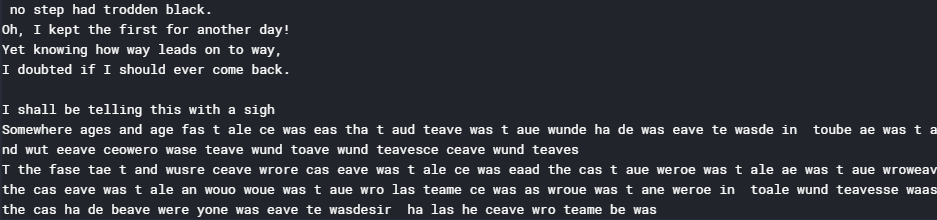# ML | Text Generation using Gated Recurrent Unit Networks

This article will demonstrate how to build a Text Generator by building a Gated Recurrent Unit Network. The conceptual procedure of training the network is to first feed the network a mapping of each character present in the text on which the network is training to a unique number. Each character is then hot-encoded into a vector which is the required format for the network.

The data for the described procedure is a collection of short and famous poems by famous poets and is in a .txt format. It can be downloaded from here.

Step 1: Importing the required libraries

 `from` `__future__ ``import` `absolute_import, division, ` `                       ``print_function, unicode_literals ` ` `  `import` `numpy as np ` `import` `tensorflow as tf ` ` `  `from` `keras.models ``import` `Sequential ` `from` `keras.layers ``import` `Dense, Activation ` `from` `keras.layers ``import` `LSTM ` ` `  `from` `keras.optimizers ``import` `RMSprop ` ` `  `from` `keras.callbacks ``import` `LambdaCallback ` `from` `keras.callbacks ``import` `ModelCheckpoint ` `from` `keras.callbacks ``import` `ReduceLROnPlateau ` `import` `random ` `import` `sys `

 `# Changing the working location to the location of the text file ` `cd C:\Users\Dev\Desktop\Kaggle\Poems ` ` `  `# Reading the text file into a string ` `with ``open``(``'poems.txt'``, ``'r'``) as ``file``: ` `    ``text ``=` `file``.read() ` ` `  `# A preview of the text file     ` `print``(text) `Step 3: Creating a mapping from each unique character in the text to a unique number

 `# Storing all the unique characters present in the text ` `vocabulary ``=` `sorted``(``list``(``set``(text))) ` ` `  `# Creating dictionaries to map each character to an index ` `char_to_indices ``=` `dict``((c, i) ``for` `i, c ``in` `enumerate``(vocabulary)) ` `indices_to_char ``=` `dict``((i, c) ``for` `i, c ``in` `enumerate``(vocabulary)) ` ` `  `print``(vocabulary) `Step 4: Pre-processing the data

 `# Dividing the text into subsequences of length max_length ` `# So that at each time step the next max_length characters  ` `# are fed into the network ` `max_length ``=` `100` `steps ``=` `5` `sentences ``=` `[] ` `next_chars ``=` `[] ` `for` `i ``in` `range``(``0``, ``len``(text) ``-` `max_length, steps): ` `    ``sentences.append(text[i: i ``+` `max_length]) ` `    ``next_chars.append(text[i ``+` `max_length]) ` `     `  `# Hot encoding each character into a boolean vector ` ` `  `# Initializing a matrix of boolean vectors with each column representing ` `# the hot encoded representation of the character ` `X ``=` `np.zeros((``len``(sentences), max_length, ``len``(vocabulary)), dtype ``=` `np.``bool``) ` `y ``=` `np.zeros((``len``(sentences), ``len``(vocabulary)), dtype ``=` `np.``bool``) ` ` `  `# Placing the value 1 at the appropriate position for each vector ` `# to complete the hot-encoding process ` `for` `i, sentence ``in` `enumerate``(sentences): ` `    ``for` `t, char ``in` `enumerate``(sentence): ` `        ``X[i, t, char_to_indices[char]] ``=` `1` `    ``y[i, char_to_indices[next_chars[i]]] ``=` `1`

Step 5: Building the GRU network

 `# Initializing the LSTM network ` `model ``=` `Sequential() ` ` `  `# Defining the cell type ` `model.add(GRU(``128``, input_shape ``=``(max_length, ``len``(vocabulary)))) ` ` `  `# Defining the densely connected Neural Network layer ` `model.add(Dense(``len``(vocabulary))) ` ` `  `# Defining the activation function for the cell ` `model.add(Activation(``'softmax'``)) ` ` `  `# Defining the optimizing function ` `optimizer ``=` `RMSprop(lr ``=` `0.01``) ` ` `  `# Configuring the model for training ` `model.``compile``(loss ``=``'categorical_crossentropy'``, optimizer ``=` `optimizer) `

Step 6: Defining some helper functions which will be used during the training of the network

Note that the first two functions given below have been referred from the documentation of the official text generation example from the Keras team.

a) Helper function to sample the next character:

 `# Helper function to sample an index from a probability array ` `def` `sample_index(preds, temperature ``=` `1.0``): ` `# temperature determines the freedom the function has when generating text ` ` `  `    ``# Converting the predictions vector into a numpy array ` `    ``preds ``=` `np.asarray(preds).astype(``'float64'``) ` ` `  `    ``# Normalizing the predicitons array ` `    ``preds ``=` `np.log(preds) ``/` `temperature ` `    ``exp_preds ``=` `np.exp(preds) ` `    ``preds ``=` `exp_preds ``/` `np.``sum``(exp_preds) ` ` `  `    ``# The main sampling step. Creates an array of probablities signifying ` `    ``# the probability of each character to be the next character in the  ` `    ``# generated text ` `    ``probas ``=` `np.random.multinomial(``1``, preds, ``1``) ` ` `  `    ``# Returning the character with maximum probability to be the next character ` `    ``# in the generated text ` `    ``return` `np.argmax(probas) `

b) Helper function to generate text after each epoch

 `# Helper function to generate text after the end of each epoch ` `def` `on_epoch_end(epoch, logs): ` `    ``print``() ` `    ``print``(``'----- Generating text after Epoch: % d'` `%` `epoch) ` ` `  `    ``# Choosing a random starting index for the text generation ` `    ``start_index ``=` `random.randint(``0``, ``len``(text) ``-` `max_length ``-` `1``) ` ` `  `    ``# Sampling for different values of diversity ` `    ``for` `diversity ``in` `[``0.2``, ``0.5``, ``1.0``, ``1.2``]: ` `        ``print``(``'----- diversity:'``, diversity) ` ` `  `        ``generated ``=` `'' ` ` `  `        ``# Seed sentence ` `        ``sentence ``=` `text[start_index: start_index ``+` `max_length] ` ` `  `        ``generated ``+``=` `sentence ` `        ``print``(``'----- Generating with seed: "'` `+` `sentence ``+` `'"'``) ` `        ``sys.stdout.write(generated) ` ` `  `        ``for` `i ``in` `range``(``400``): ` `            ``# Initializing the predicitons vector ` `            ``x_pred ``=` `np.zeros((``1``, max_length, ``len``(vocabulary))) ` ` `  `            ``for` `t, char ``in` `enumerate``(sentence): ` `                ``x_pred[``0``, t, char_to_indices[char]] ``=` `1.` ` `  `            ``# Making the predictions for the next character ` `            ``preds ``=` `model.predict(x_pred, verbose ``=` `0``)[``0``] ` ` `  `            ``# Getting the index of the most probable next character ` `            ``next_index ``=` `sample_index(preds, diversity) ` ` `  `            ``# Getting the most probable next character using the mapping built ` `            ``next_char ``=` `indices_to_char[next_index] ` ` `  `            ``# Building the generated text ` `            ``generated ``+``=` `next_char ` `            ``sentence ``=` `sentence[``1``:] ``+` `next_char ` ` `  `            ``sys.stdout.write(next_char) ` `            ``sys.stdout.flush() ` `        ``print``() ` ` `  `# Defining a custom callback function to  ` `# describe the internal states of the network ` `print_callback ``=` `LambdaCallback(on_epoch_end ``=` `on_epoch_end) `

c) Helper function to save the model after each epoch in which loss decreases

 `# Defining a helper function to save the model after each epoch ` `# in which the loss decreases ` `filepath ``=` `"weights.hdf5"` `checkpoint ``=` `ModelCheckpoint(filepath, monitor ``=``'loss'``, ` `                             ``verbose ``=` `1``, save_best_only ``=` `True``, ` `                             ``mode ``=``'min'``) `

d) Helper function to reduce the learning rate each time the learning plateaus

 `# Defining a helper function to reduce the learning rate each time ` `# the learning plateaus ` `reduce_alpha ``=` `ReduceLROnPlateau(monitor ``=``'loss'``, factor ``=` `0.2``, ` `                              ``patience ``=` `1``, min_lr ``=` `0.001``) ` `callbacks ``=` `[print_callback, checkpoint, reduce_alpha] `

Step 7: Training the GRU model

 `# Training the GRU model ` `model.fit(X, y, batch_size ``=` `128``, epochs ``=` `30``, callbacks ``=` `callbacks) `Step 8: Generating new and random text

 `def` `generate_text(length, diversity): ` `    ``# Get random starting text ` `    ``start_index ``=` `random.randint(``0``, ``len``(text) ``-` `max_length ``-` `1``) ` ` `  `    ``# Defining the generated text ` `    ``generated ``=` `'' ` `    ``sentence ``=` `text[start_index: start_index ``+` `max_length] ` `    ``generated ``+``=` `sentence ` ` `  `    ``# Generating new text of given length ` `    ``for` `i ``in` `range``(length): ` ` `  `            ``# Initializing the predicition vector ` `            ``x_pred ``=` `np.zeros((``1``, max_length, ``len``(vocabulary))) ` `            ``for` `t, char ``in` `enumerate``(sentence): ` `                ``x_pred[``0``, t, char_to_indices[char]] ``=` `1.` ` `  `            ``# Making the predicitons ` `            ``preds ``=` `model.predict(x_pred, verbose ``=` `0``)[``0``] ` ` `  `            ``# Getting the index of the next most probable index ` `            ``next_index ``=` `sample_index(preds, diversity) ` ` `  `            ``# Getting the most probable next character using the mapping built ` `            ``next_char ``=` `indices_to_char[next_index] ` ` `  `            ``# Generating new text ` `            ``generated ``+``=` `next_char ` `            ``sentence ``=` `sentence[``1``:] ``+` `next_char ` `    ``return` `generated ` ` `  `print``(generate_text(``500``, ``0.2``)) `Note: Although the output does not make much sense now, the output can be significantly improved by training the model for more epochs.

Attention geek! Strengthen your foundations with the Python Programming Foundation Course and learn the basics.

To begin with, your interview preparations Enhance your Data Structures concepts with the Python DS Course.

My Personal Notes arrow_drop_upCheck out this Author's contributed articles.

If you like GeeksforGeeks and would like to contribute, you can also write an article using contribute.geeksforgeeks.org or mail your article to contribute@geeksforgeeks.org. See your article appearing on the GeeksforGeeks main page and help other Geeks.

Please Improve this article if you find anything incorrect by clicking on the "Improve Article" button below.

Article Tags :
Practice Tags :

Be the First to upvote.

Please write to us at contribute@geeksforgeeks.org to report any issue with the above content.